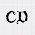# C# NullReferenceException : Object reference not set to an instance of an object

In simple words this error means that you are trying to use a reference of an object which is null i.e. you haven't initialized it yet. If you have to use any object you have to first initialize it. This error is a run time exception.

For example :

string str = null;
int length = str.Length; // This line will give you NullReferenceException because there is no string (string is null) from where you can get a length.

.Net variables are of two types, value types and reference types. Value types (i.e. Integers, Booleans etc) when declared are automatically initialized with their default value. Integers with 0 and booleans's with false. That means if you are declaring integer or boolean values you are actually initializing them with default value. But, reference type when declared do not have default value.

public class Shape {
public int Length {get;set;}
}
public class Square {
public Shape ShapeOfSquare {get;set;}
}

Square sq = new Square();

int lengthOfSquare = sq.ShapeOfSquare.Length;

So in the above example you have only declared ShapeOfSquare but not initialized it, and this is a reference type so it will not have a default value. Thus you will get NullReferenceException while executing the above code.

In arrays or collections also if you haven't initialized them and trying to access any value using an index it will give you NullReferenceException. For example,

int[] values = null;
int number = value;

values is null, so there is no array from where you can get a value.

When you are casting a null object into a value type, this will also gives you the same exception. For example, the code below will throw a NullReferenceException

object obj = null;
int value = (Int32)obj;

How to avoid NullReferenceException error.

You can check for null values and ignore them. Suppose we have the same example which gives us NullReferenceException. So we should first check if that object is null or not.

public class Shape {
public int Length {get;set;}
}
public class Square {
public Shape ShapeOfSquare {get;set;}
}

Square sq = new Square();

if(sq != null)
{
int lengthOfSquare = sq.ShapeOfSquare.Length;
}

If any variable is null you can even give a defualt value to that variable, so that it will not give you a runtime exception.

For variable of string type you can check if the string is null or not using a function IsNullOrWhiteSpace() or IsNullOrEmpty().

If you have any questions, feel free to ask.

1.when we set the value error same error genrated. why ?
sq.ShapeOfSquare.Length=10;

1.Even if you set the value using sq.ShapeOfSquare.Length = 10, ShapeOfSquare is still a reference type and it is not initialized.

2.Sir,

Please tell how to initialize a class?

2.